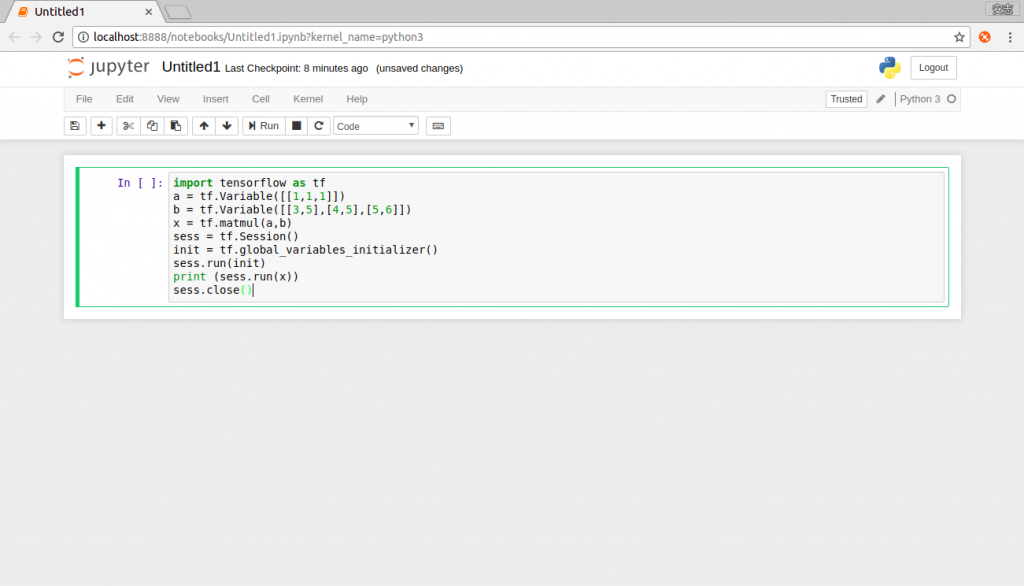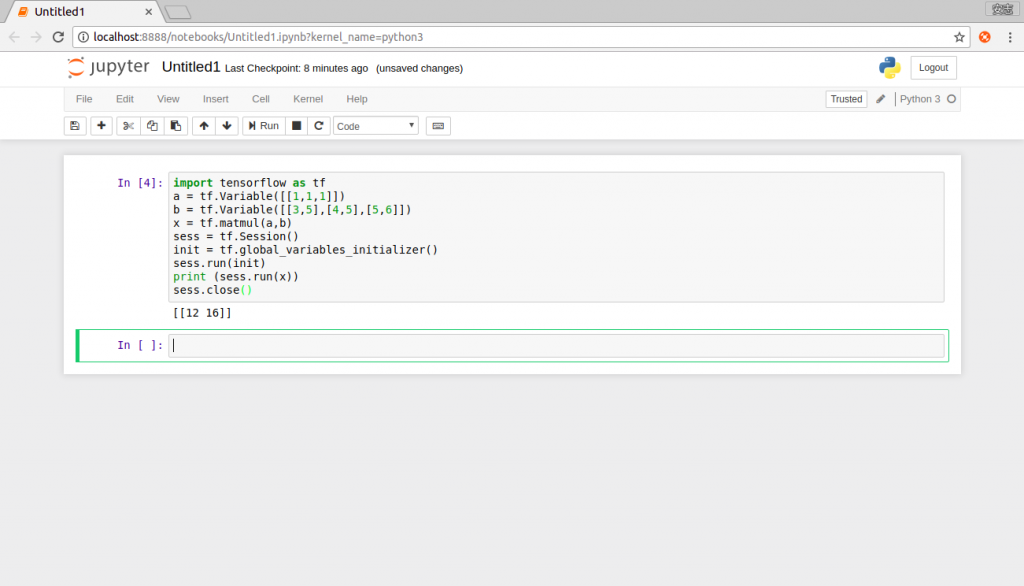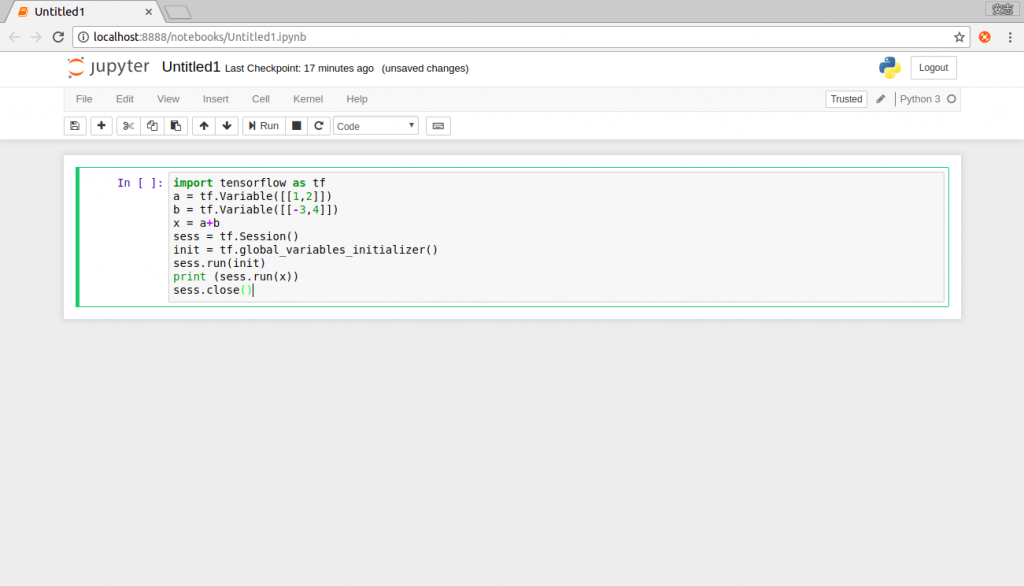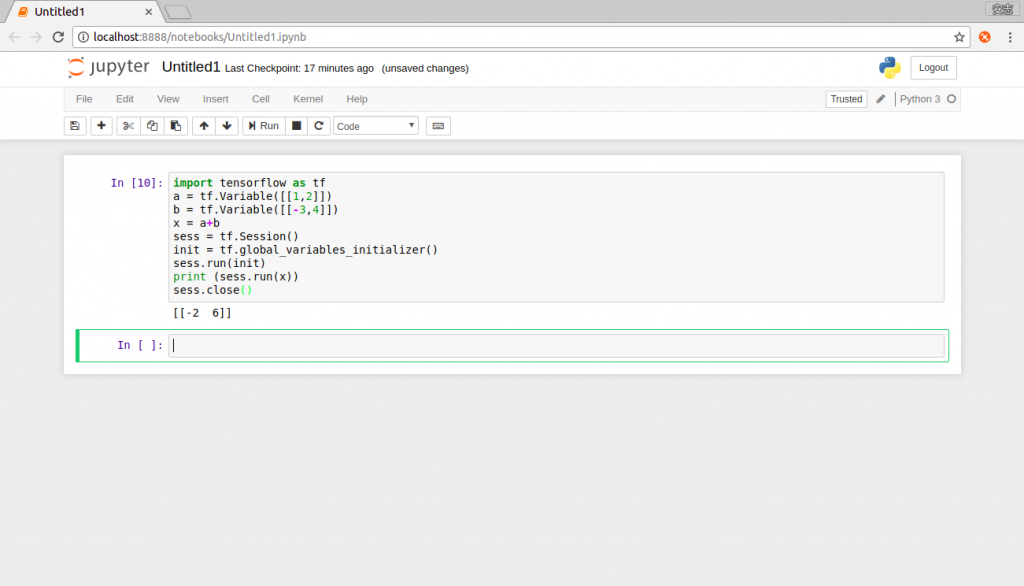#2018 iT 邦幫忙鐵人賽DAY 13
0
AI & Machine Learning

# 前言：

2維的Tensor(張量)就是矩陣，所以矩陣的基本運算再tensorflow中是十分重要的，而再資訊工程中有一門必修就是線性代數，再那裡面會教到很多矩陣的東西，但因為是2年前的事情了，所以筆者記憶有點模糊了，但就加減複習一下吧。

# 程式開始：

## 相乘：

``````import tensorflow as tf
a = tf.Variable([[1,1,1]])
b = tf.Variable([[3,5],[4,5],[5,6]])
x = tf.matmul(a,b)
sess = tf.Session()
init = tf.global_variables_initializer()
sess.run(init)
print (sess.run(x))
sess.close()
``````

### 答案：

[(1x3+1x4+1x5) (1x5+1x5+1x6)]=[12 16]

### 執行結果：## 相加：

``````import tensorflow as tf
a = tf.Variable([[1,2]])
b = tf.Variable([[-3,4]])
x = a+b
sess = tf.Session()
init = tf.global_variables_initializer()
sess.run(init)
print (sess.run(x))
sess.close()
``````

### 答案：

[(1+(-3)) (2+4)] = [-2 6]

### 執行結果：